# Absolute Value Inequalities

Absolute Value Inequalities

An absolute value inequality is an inequality with an absolute value symbol in it.

It can be solved by two methods.

1. Using number line
2. Using formulas

Once you know these methods, you can solve absolute value inequalities problems with or without an absolute value inequalities calculator.

Check out the interactive simulations to know more about the lesson and try your hand at solving a few interesting practice questions at the end of the page.

## Lesson Plan

 1 What Are Absolute Value Inequalities? 2 Important Notes on Absolute Value Inequalities 3 Tips and Tricks 4 Solved Examples on Absolute Value Inequalities 5 Interactive Problems on Absolute Value Inequalities

## What Are Absolute Value Inequalities?

An absolute value inequality is an inequality which involves an absolute value expression with variables.

That is, an absolute value inequality can be one of the following forms (or) can be converted to one of the following forms:

 \begin{align} |ax+b| &< c\0.2cm] |ax+b|&>c \\[0.2cm] |ax+b| &\leq c\\[0.2cm] |ax+b| &\geq c \end{align} So the absolute value inequalities are of two types: • one with $$<$$ or $$\leq$$ • one with $$>$$ or $$\geq$$ We will study about each type of inequalities in detail. ## How to Solve Absolute Value Inequalities? Here is the procedure of solving any type of absolute value inequality using the number line. The procedure is shown step-by-step along with an example. Example Solve the absolute value inequality $$|x+2| < 4$$ Step 1: Assume the inequality as an equation and solve it. Convert the inequality sign "<" in our inequality to "=" and solve it. \[|x+2|=4

Removing the absolute value sign on the left side, we get $$\pm$$ sign on the other side.

$x+2=\pm 4$

This results in two equations, one with "+" and the other with "-".

\begin{align} x+2 &=4 & x+2 &=-4\\[0.2cm] x&=2 & x&=-6 \end{align}

Step 2: Represent the solutions from Step 1 on a number line in order.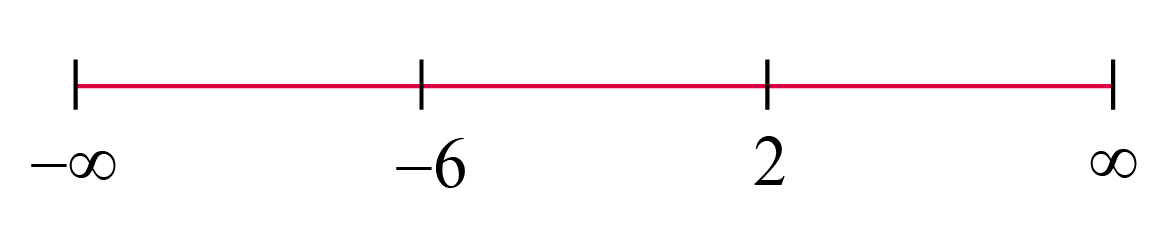Here, we can see that the number line is divided into 3 parts.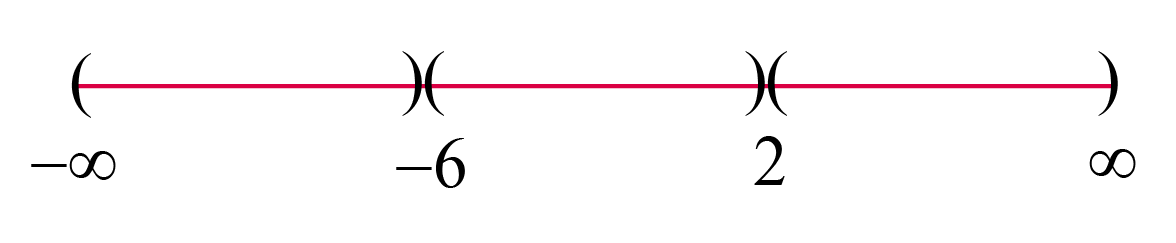Step 3: Take a random number from each of these intervals and substitute it in the given inequality.

Identify which of these numbers actually satisfy the given inequality.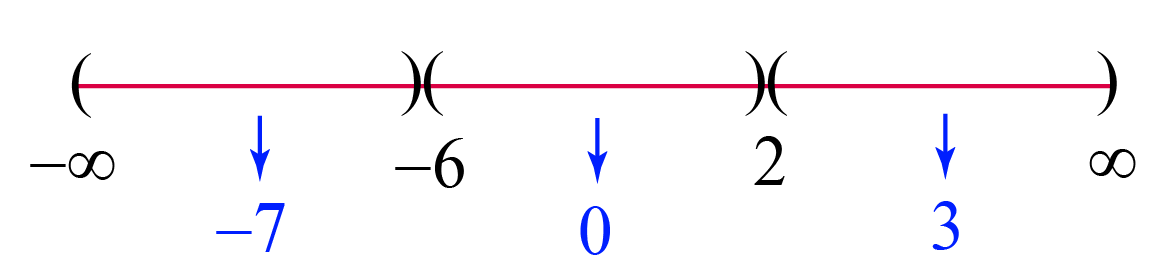Interval

Random Number Checking the given inequality with random number
$$(-\infty, -6)$$ -7 \begin{align} |-7+2|<4\\[0.2cm] 5<4\\[0.2cm] \text{This is False} \end{align}
$$(-6, 2)$$ 0 \begin{align} |0+2|<4\\[0.2cm] 2<4\\[0.2cm] \text{This is True} \end{align}
$$(2, \infty)$$ 3 \begin{align} |3+2|<4\\[0.2cm] 5<4\\[0.2cm] \text{This is False} \end{align}

Step 4: The solution of the given inequality is the interval(s) which lead to True in the above table

Therefore, the solution of the given inequality is,

 $$(-6,2)$$ (or) $$-6 This procedure is summarized in the following flowchart.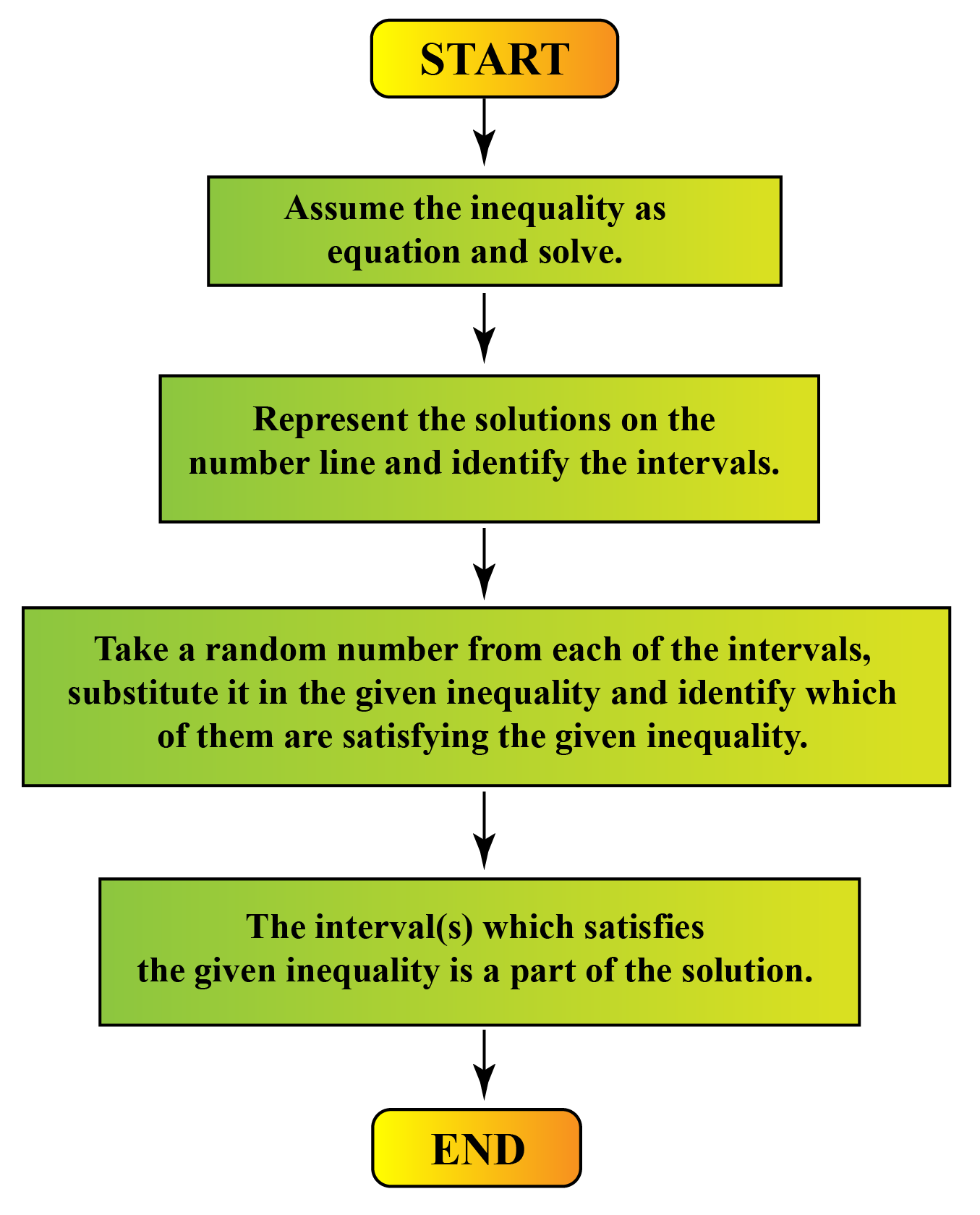Note: 1. If the problem was \(|x+2| \leq 4$$, then the solution would have been $$[-6, 2]$$ (or) $$-6\leq x \leq 2$$. i.e.,$\begin{array}{l}\text { If }|x+2|<4 \Rightarrow (-6, 2) \text { OR } -6 < x <2 \\\text { If }|x+2| \leq \Rightarrow [-6, 2] \text{ OR } -6 \leq x \leq 2\end{array}$
2.  Also,$$\text{If} |x+2|\!>\!4\Rightarrow(-\infty,-6)\cup(2, \infty)$$
$$\text{(OR)}(-\infty<x<-6)\cup(2<x<\infty)$$

$$\text { If }|x+2| \geq 4 \Rightarrow(-\infty,-6] \cup[2, \infty)$$
$$\text{ (OR) }(-\infty<x \leq-6) \cup(2\leq x<\infty)$$

The same trick is explained in the following "Important Notes."

## Graphing Absolute Value Inequalities

While graphing absolute inequalities, we have to keep the following things in mind.

• Use open dots at the endpoints of the open intervals (i.e. the intervals like $$(a,b$$).
• Use closed dots at the endpoints of the closed intervals (i.e. the intervals like $$[a,b]$$).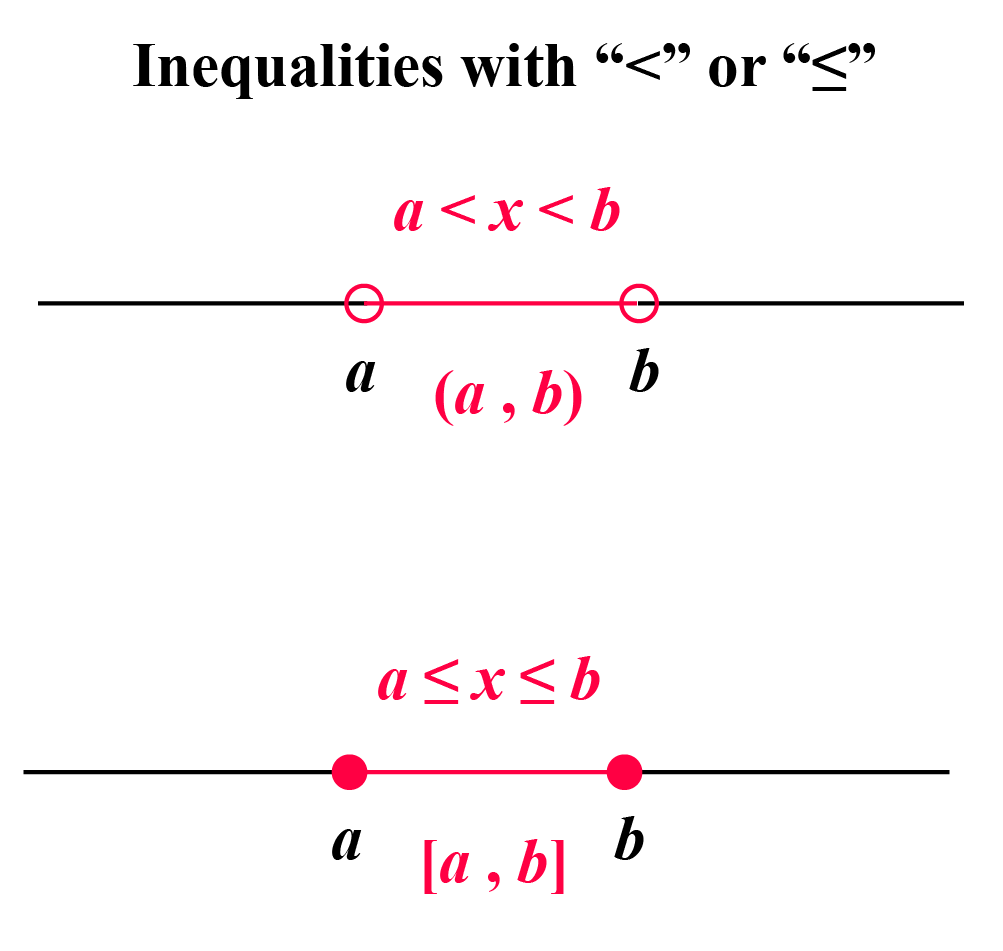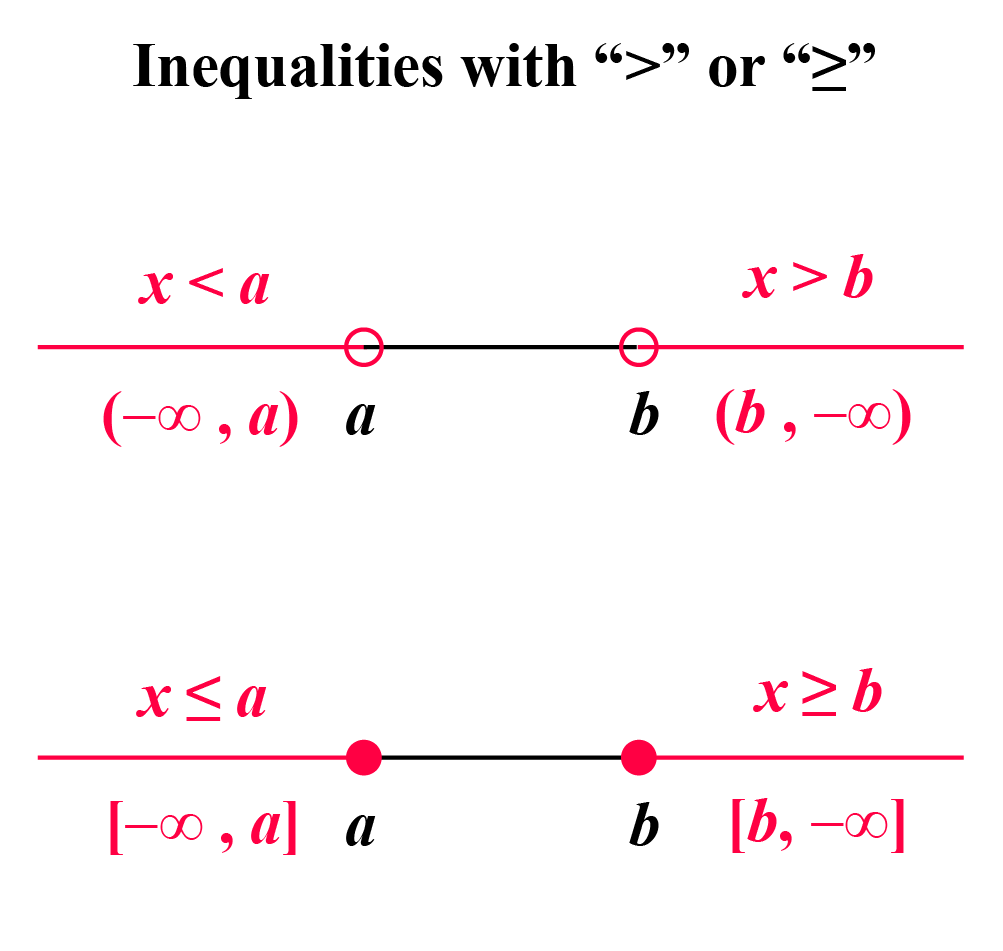## General Formula of Absolute Value Inequalities

So far we have learned the procedure of solving the absolute value inequalities using the number line.

This procedure works for any type of inequality.

In fact, inequalities can be solved using formulas as well.

To apply the formulas, first, we need to isolate the absolute value expression on the left side of the inequality.

There are 4 cases to remember for solving the inequalities using the formulas.

Let us assume that $$a$$ is a positive real number in all the cases.

### Case 1: When the Inequality Is of the Form:

$|x|<a \text { or }|x| \leq a$

In this case, we use the following formulas to solve the inequality:

### Case 3: When the Inequality Is of the Form:

$|x| <-a \text { or } |x| \leq-a$

We know that the absolute value always results in a positive value.

Thus $$|x|$$ is always positive.

Also, $$-a$$ is negative (as we assumed $$a$$ is positive).

Thus the above two inequalities mean that "positive number is less than (or less than or equal to) negative number," which is never true.

Thus, all such inequalities have no solution.

 \begin{aligned} |x|<-a &\text { or } |x| \leq-a \0.2cm]&\Rightarrow \\[0.2cm] \text{ No } & \text{Solution} \end{aligned} ### Case 4: When the Inequality Is of the Form: \[|x| >-a \text { or } |x| \geq-a

We know that the absolute value always results in a positive value.

Thus $$|x|$$ is always positive.

Also, $$-a$$ is negative (as we assumed $$a$$ is positive).

Thus the above two inequalities mean that "positive number is greater than (or greater than or equal to) negative number," which is always true.

Thus, the solution of all such inequalities is the set of all real numbers, $$R$$.

 \begin{aligned} |x|>-a &\text { or } |x| \geq-a \0.2cm]&\Rightarrow \\[0.2cm] \text{ Set of all } & \text{ Real Numbers, R} \end{aligned} All these formulas of absolute value inequalities are summarized in the following flowchart.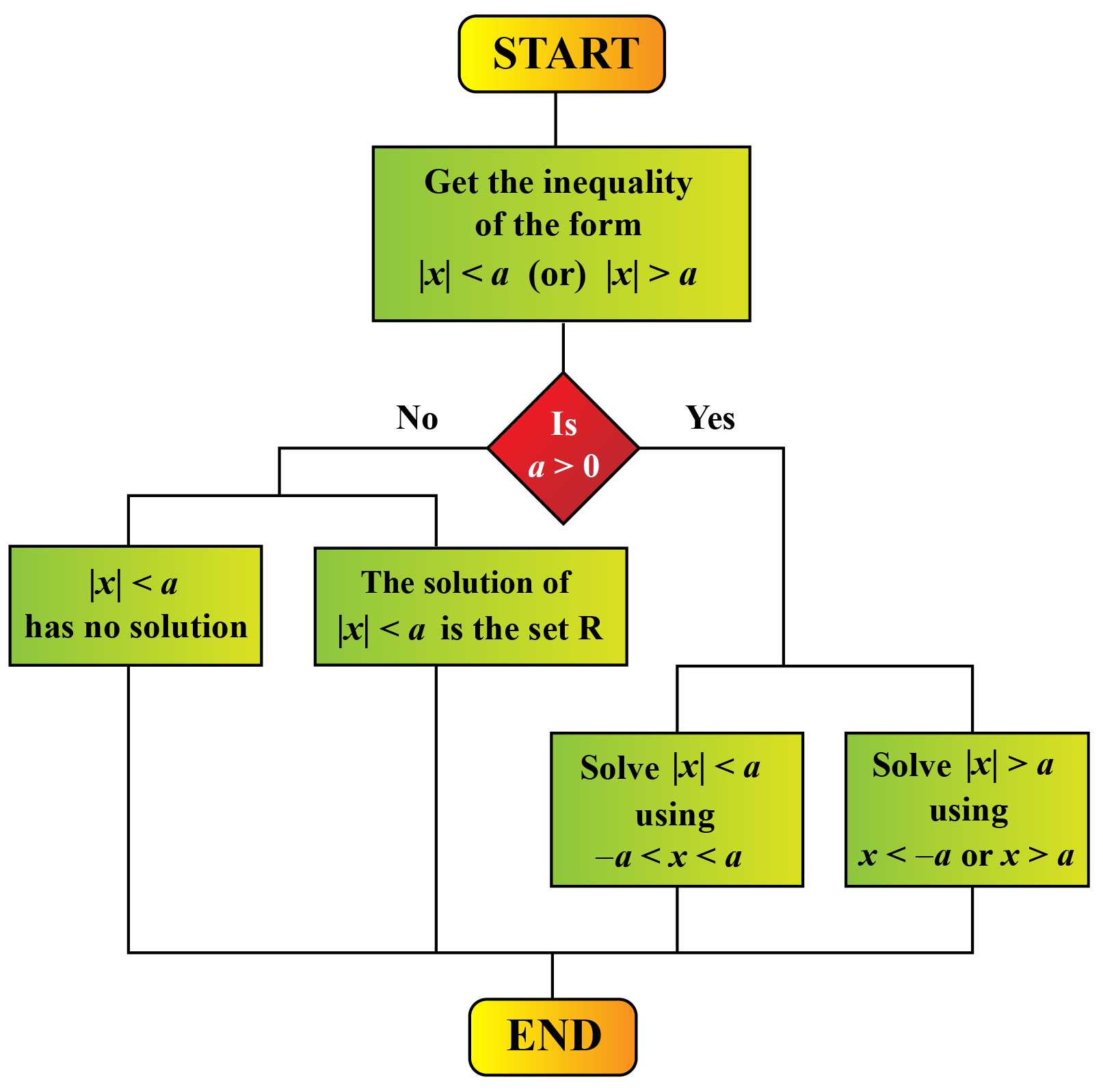You can find absolute value inequalities examples under the "Solved Examples" section of this page.Tips and Tricks After we get the inequality to the form $$|x| \text{ inequality symbol }a$$, if $$a$$ is negative, then we don't need to solve it by any method. Straight away, we can write the answer using the following tricks. 1. If the inequality symbol is $$<$$ or $$\leq$$, then the inequality has "no solution." 2. If the inequality symbol is $$>$$ or $$\geq$$, then the solution is the "set of all real numbers, $$R$$." ## Absolute Value Inequalities Calculator Here is the "Absolute Value Inequalities Calculator." It allows you to find the solution of any absolute value inequality and shows the solution in 3 forms: inequality form, interval form, and graphical form. ## Solved Examples Here are absolute value inequalities problems.  Example 1 Mark is asked to solve the following absolute value inequality and asked to write the solution in 3 forms. 1. Inequality form 2. Interval form 3. Graphical form Can we help him in finding the solution? \[|2 x-1|-9 \geq-5

Also, show him the solution on the number line.

Solution

The given absolute value inequality is:

$|2 x-1|-9 \geq-5$

First, we need to isolate the absolute value expression.

For that, we will add 9 on both sides. Then we get:

$|2 x-1| \geq 4$

Using the formulas we learned:

\begin{align} &2 x-1 \leq-4 \text{ (or) }2 x-1 \geq 4\\[0.2cm] &\text { Adding 1 on both sides }\\[0.2cm] &2 x \leq-3 \text{ (or) } 2 x \geq 5 \\[0.2cm] &\text { Dividing both sides by } 2,\\[0.2cm] &x \leq-\frac{3}{2} \text{ (or) } x \geq \frac{5}{2} \end{align}

Therefore, the solution is:

 $$x \leq-\frac{3}{2} \text{ (or) } x \geq, \frac{5}{2}$$ (OR) $$\left(-\infty,-\frac{3}{2}\right)\cup\left(\frac{5}{2}, \infty\right)$$

Let us represent the solution on the graph.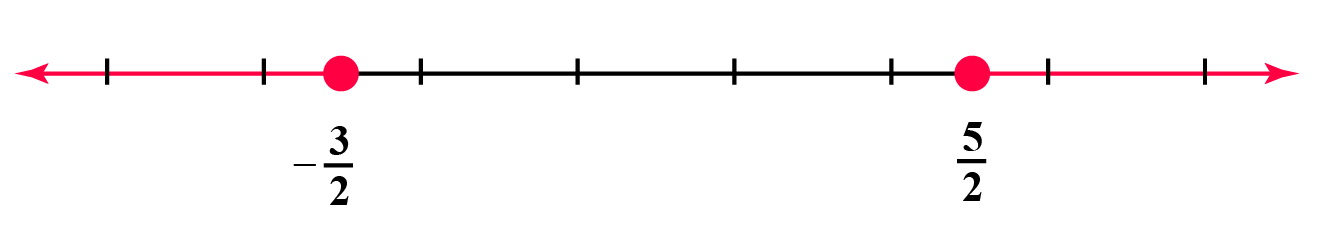Example 2

A carpenter is making a handcrafted table. In order for the leg to fit, it needs to be 250 millimeters wide, allowing for a margin of error of 3.5 millimeters.

Write an absolute value inequality that models this relationship, and then find the range of the table leg's widths.

Solution

Let us assume that the width of the table leg to be $$x$$.

Then the absolute value inequality that corresponds to the given scenario is,

$|x-250| \leq 3.5$

Using the formulas we learned:

\begin{align} &-3.5 \leq x-250 \leq 3.5\\[0.2cm] & \text{Adding 250 on all the sides,}\\[0.2cm] &246.5\leq x \leq 253.5 \end{align}

The range of the table leg's width is:

 $$[246.5,253.5]$$ (in mm)
 Example 3

Before a piece of steel can be sold for its maximum price, it must be 50 feet long with an absolute error of 2 feet.

Find the range of acceptable heights for pieces of steel that are to be sold at full price by writing an absolute value inequality to represent this situation and then solving it.

Solution

Let us assume that the height of the piece of steel is $$x$$ feet.

Then the absolute value inequality that corresponds to the given scenario is,

$|x-50| \leq 2$

Using the formulas we learned:

\begin{align} &-2 \leq x-50 \leq 2\\[0.2cm] & \text{Adding 50 on all the sides,}\\[0.2cm] &48 \leq x \leq 52\end{align}

The range of the acceptable heights of steels is:

 $$[48, 52]$$ (in feet)

## Practice Problems

Here are a few activities for you to practice. Select/Type your answer and click the "Check Answer" button to see the result.

## Let's Summarize

We hope you enjoyed learning about Absolute Value Inequalities with the simulations and practice questions. Now you will be able to easily solve problems on absolute value inequalities problems, absolute value inequalities calculator, and absolute value inequalities examples.

At Cuemath, our team of math experts is dedicated to making learning fun for our favorite readers, the students!

Through an interactive and engaging learning-teaching-learning approach, the teachers explore all angles of a topic.

Be it worksheets, online classes, doubt sessions, or any other form of relation, it’s the logical thinking and smart learning approach that we, at Cuemath, believe in.

## 1. Where can I find the absolute value inequalities calculator?

You can find the "absolute value inequalities calculator" on this page itself.

## 2. How to graph absolute value inequalities?

While graphing the absolute inequalities, we have to keep the following things in mind.

• Use open dots at the endpoints of the open intervals (i.e. the intervals like $$(a,b$$).
• Use closed dots at the endpoints of the closed intervals (i.e. the intervals like $$[a,b]$$).

More Important Topics
Numbers
Algebra
Geometry
Measurement
Money
Data
Trigonometry
Calculus
More Important Topics
Numbers
Algebra
Geometry
Measurement
Money
Data
Trigonometry
Calculus